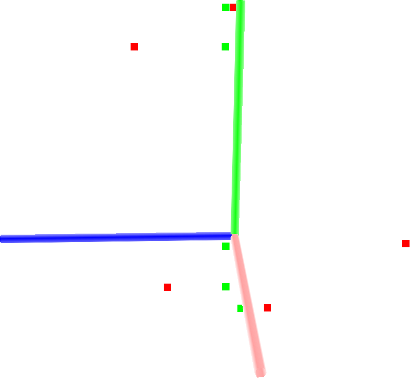# Projecting points using a parametric model

In this tutorial we will learn how to project points onto a parametric model (e.g., plane, sphere, etc). The parametric model is given through a set of coefficients – in the case of a plane, through its equation: ax + by + cz + d = 0.

# The code

First, create a file, let’s say, `project_inliers.cpp` in your favorite editor, and place the following inside it:

``` 1#include <iostream>
2#include <pcl/point_cloud.h> // for PointCloud
3#include <pcl/point_types.h>
4#include <pcl/ModelCoefficients.h>
5#include <pcl/filters/project_inliers.h>
6
7int
8 main ()
9{
10  pcl::PointCloud<pcl::PointXYZ>::Ptr cloud (new pcl::PointCloud<pcl::PointXYZ>);
11  pcl::PointCloud<pcl::PointXYZ>::Ptr cloud_projected (new pcl::PointCloud<pcl::PointXYZ>);
12
13  // Fill in the cloud data
14  cloud->width  = 5;
15  cloud->height = 1;
16  cloud->points.resize (cloud->width * cloud->height);
17
18  for (auto& point: *cloud)
19  {
20    point.x = 1024 * rand () / (RAND_MAX + 1.0f);
21    point.y = 1024 * rand () / (RAND_MAX + 1.0f);
22    point.z = 1024 * rand () / (RAND_MAX + 1.0f);
23  }
24
25  std::cerr << "Cloud before projection: " << std::endl;
26  for (const auto& point: *cloud)
27    std::cerr << "    " << point.x << " "
28                        << point.y << " "
29                        << point.z << std::endl;
30
31  // Create a set of planar coefficients with X=Y=0,Z=1
32  pcl::ModelCoefficients::Ptr coefficients (new pcl::ModelCoefficients ());
33  coefficients->values.resize (4);
34  coefficients->values = coefficients->values = 0;
35  coefficients->values = 1.0;
36  coefficients->values = 0;
37
38  // Create the filtering object
39  pcl::ProjectInliers<pcl::PointXYZ> proj;
40  proj.setModelType (pcl::SACMODEL_PLANE);
41  proj.setInputCloud (cloud);
42  proj.setModelCoefficients (coefficients);
43  proj.filter (*cloud_projected);
44
45  std::cerr << "Cloud after projection: " << std::endl;
46  for (const auto& point: *cloud_projected)
47    std::cerr << "    " << point.x << " "
48                        << point.y << " "
49                        << point.z << std::endl;
50
51  return (0);
52}
```

# The explanation

Now, let’s break down the code piece by piece.

We first import the ModelCoefficients structure then the ProjectInliers filter.

```#include <pcl/ModelCoefficients.h>
#include <pcl/filters/project_inliers.h>
```

We then create the point cloud structure, fill in the respective values, and display the content on screen.

```  cloud->width  = 5;
cloud->height = 1;
cloud->points.resize (cloud->width * cloud->height);

for (auto& point: *cloud)
{
point.x = 1024 * rand () / (RAND_MAX + 1.0f);
point.y = 1024 * rand () / (RAND_MAX + 1.0f);
point.z = 1024 * rand () / (RAND_MAX + 1.0f);
}

std::cerr << "Cloud before projection: " << std::endl;
for (const auto& point: *cloud)
std::cerr << "    " << point.x << " "
<< point.y << " "
<< point.z << std::endl;
```

We fill in the ModelCoefficients values. In this case, we use a plane model, with ax+by+cz+d=0, where a=b=d=0, and c=1, or said differently, the X-Y plane.

```  pcl::ModelCoefficients::Ptr coefficients (new pcl::ModelCoefficients ());
coefficients->values.resize (4);
coefficients->values = coefficients->values = 0;
coefficients->values = 1.0;
coefficients->values = 0;
```

We create the ProjectInliers object and use the ModelCoefficients defined above as the model to project onto.

```  pcl::ProjectInliers<pcl::PointXYZ> proj;
proj.setModelType (pcl::SACMODEL_PLANE);
proj.setInputCloud (cloud);
proj.setModelCoefficients (coefficients);
proj.filter (*cloud_projected);
```

Finally we show the content of the projected cloud.

```  std::cerr << "Cloud after projection: " << std::endl;
for (const auto& point: *cloud_projected)
std::cerr << "    " << point.x << " "
<< point.y << " "
<< point.z << std::endl;
```

# Compiling and running the program

``` 1cmake_minimum_required(VERSION 3.5 FATAL_ERROR)
2
3project(project_inliers)
4
5find_package(PCL 1.2 REQUIRED)
6
7include_directories(\${PCL_INCLUDE_DIRS})
10
```

After you have made the executable, you can run it. Simply do:

```\$ ./project_inliers
```

You will see something similar to:

```Cloud before projection:
0.352222 -0.151883 -0.106395
-0.397406 -0.473106 0.292602
-0.731898 0.667105 0.441304
-0.734766 0.854581 -0.0361733
-0.4607 -0.277468 -0.916762
Cloud after projection:
0.352222 -0.151883 0
-0.397406 -0.473106 0
-0.731898 0.667105 0
-0.734766 0.854581 0
-0.4607 -0.277468 0
```

A graphical display of the projection process is shown below.Note that the coordinate axes are represented as red (x), green (y), and blue (z). The five points are represented with red as the points before projection and green as the points after projection. Note that their z now lies on the X-Y plane.